# scipy.spatial.distance.dice¶

scipy.spatial.distance.dice(u, v)

Computes the Dice dissimilarity between two boolean n-vectors u and v, which is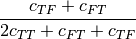where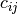is the number of occurrences of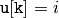and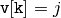for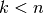.

Parameters : u : ndarray An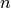-dimensional vector. v : ndarray An-dimensional vector. d : double The Dice dissimilarity between vectors u and v.

#### Previous topic

scipy.spatial.distance.cosine

#### Next topic

scipy.spatial.distance.euclidean